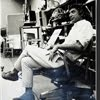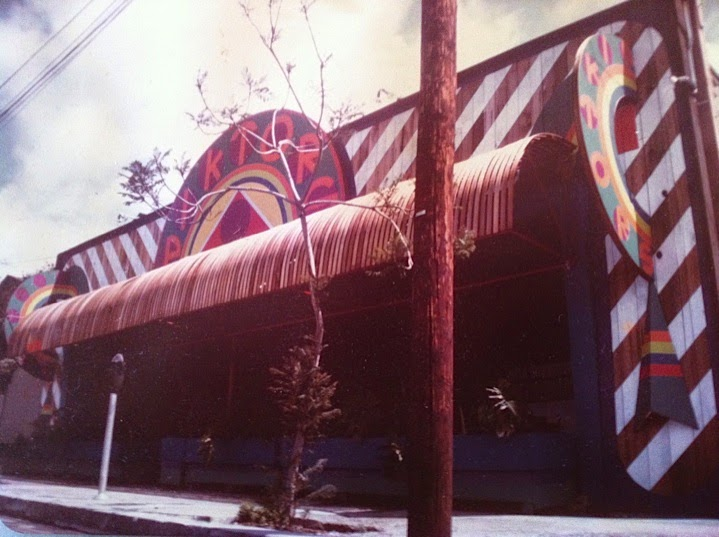## Eugene Ray SDSU 1970'sEugene Ray 1970's. photo credit: Tom Davis

## Friday, June 6, 2014

### HORUS THIRD EYE / vision & perception

+EXOTIC+
>is a favorite word of mine even though<
>the correct meaning is merely"foreign"<
^^^^^^^^^^^^^^^^^^^^^^^^
+EXOTIC TO ME MEANS+
>matisse's "egyptian curtain" fits my<
>perspective as well as the french+
+TROPIC SYNDROME &+
+EGYPTIAN THIRD EYE+
^^^^^^^^^^^^^^^^^^^
>yes, the french produced stamps in<
+EGYPT=MEXICO+
+INDIA=GABON+
>where a small boy in baton rouge<
>recognized the "exotic" designs<
^^^^^^^^^^^^^^^^^^^^
>christopher dunn has done a masterful job<
>to prove the technical ability of egyptian<
+(EGYPT LIKE SUMERIA+
>stone cutting was not of this planet earth<
^^^^^^^^^^^^^^^^^^^^^^^^^^^
>greek and roman architecture taught in the<
>"bannister fletcher"college ref and used so<
+(AKHENATEN was+
+"COSMIC EXOTIC")+
>well by h.h. richardson was quite polite,
>compared to the very exotic egyptian<
^^^^^^^^^^^^^^^^^^^^^^^^
>the egyptian horus "third eye" of perception<
>beyond ordinary sight was a symbol i used<
+(HORUS EYE SYMBOL /+
+RAY NOLA STUDIO-66)+
>when i opened my studio in new orleans<
>(published in the nola "item" newspaper)<
^^^^^^^^^^^^^^^^^^^^^^^^^
>the annual exhibition that i mounted at sdsu<
>made every effort to demonstrate the power<
+CHILD OF THE THIRD EYE)+
>of the artist's third eye of percept-illumens<
^^^^^^^^^^^^^^^^^^^^^^^^^>the second of three radiant concept projects<
>in the new orleans vieux carre was a child<
+WORKS REFLECT 3RD EYE)+
>of the horus third eye of artistic perception<
^^^^^^^^^^^^^^^^^^^^^^^^^^^
>the grail bookshop in paris is where one<
>goes to gather historical information of<
+(THIRD EYE DESCENT of+
+the"MARVELOUS" SHOP)+
>reference to egyptian horus third eye<
^^^^^^^^^^^^^^^^^^^^^^>the vision for pictors came to me in a flash<
>straight from the egyptian horus third eye<
+VITAL AND EXOTIC)+ !
^^^^^^^^^^^^^^^^^^^^^^^^^^^^
>san diego, california, 1975 saw the reality<
>of a horus third eye vision come to life in<
+("PICTOR'S METAMORPHOSIS"+
+/ CHILD OF THE THIRD EYE)+
>a restaurant on india street titled "pictors"<
^^^^^^^^^^^^^^^^^^^^^^^^
>matisse had a very strong horus third eye<
>and sought north africa to visit before<
+(EGYPT AGENT OF THE+
+MODERN THIRD EYE)+
>painting his famed "egyptian curtain"<
^^^^^^^^^^^^^^^^^^^^^^^^
>this is the andra ursuta sculpture that i went to<
>los ángeles to see because she was inspired by<
+(HAMMER MUSEUM DID+
+NOT SHOW THIS PIECE)+ ?
>egyptian royal mummies w/ left arm upward<
^^^^^^^^^^^^^^^^^^^^^^^^^^^
>in this case jesus is depicted as extraterrestrial<
>on an ancient coin which supports modern<
>research linking jesus to cosmic sourcing<
^^^^^^^^^^^^^^^^^^^^^^^^^^^^
>ancient archaeological images of obviously<
>alien biological entities clothed in space<
+(MANY ANCIENT PIECES+
+ARE FOUND LIKE THIS)+ !
>suits complete w/ life support equipment<
^^^^^^^^^^^^^^^^^^^^^^^^^^>the graphics of ancient egypt are filled with<
>exotic cosmic symbology linked to space<
+(GIANT SOLAR SYMBOL+
+W/ KEY OF LIFE ANKH)+
>travel and supernatural >jedi< equipment<
>(synchronistic akhenaten tv doc. tonight)<
^^^^^^^^^^^^^^^^^^^^^^^^^^
+NOTICE THE HORUS THIRD EYE+
+VISIONARY AGENT FOR THOSE+
>(diagnosed as our pineal gland)<
+WHO WANT TO SEE CLEARLY+
^^^^^^^^^^^^^^^^^^^^^^^^^
eugene ray, mfa, architect
professor emeritus, sdsu